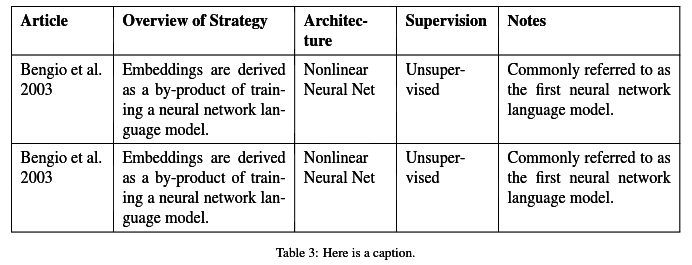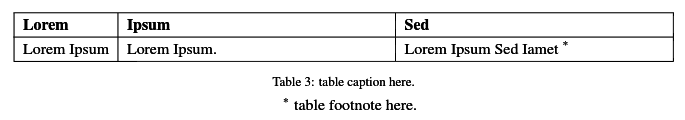LaTeX: Table Examples

# LaTeX: Table Examples

Last updated:

WIP Alert This is a work in progress. Current information is correct but more content will probably be added in the future.

## Full-width table with autosized column sizes

(using tabularx)

|L{2cm}| means left-aligned (column width will not grow past 2cm even if contents need it) and |X| means that this column will grow until the table width is reached (in this case, \fullwidth), but no more.

% custom commands
\newcolumntype{L}{>{\raggedright\let\newline\\\arraybackslash\hspace{0pt}}p{#1}}
\newcolumntype{C}{>{\centering\let\newline\\\arraybackslash\hspace{0pt}}p{#1}}
\newcolumntype{R}{>{\raggedleft\let\newline\\\arraybackslash\hspace{0pt}}p{#1}}

% this line fixes the vertical padding of text inside the cells
\renewcommand{\arraystretch}{1.4}

\begin{table*}[h]
\begin{tabularx}{\textwidth}{|L{2cm}|X|L{2cm}|L{2cm}|X|}
\hline
\textbf{Article} & \textbf{Overview of Strategy} & \textbf{Architecture} & \textbf{Supervision} & \textbf{Notes} \\ \hline
Bengio et al. 2003 & Embeddings are derived as a by-product of training a neural network language model. & Nonlinear Neural Net & Unsupervised & Commonly referred to as the first neural network language model. \\ \hline
Bengio et al. 2003 & Embeddings are derived as a by-product of training a neural network language model. & Nonlinear Neural Net & Unsupervised & Commonly referred to as the first neural network language model. \\ \hline
\end{tabularx}
\caption{Here is a caption.}
\end{table*}table produced

## Table footnotes

Using footnotes in table is cumbersome because they may end up on different pages from the one the table ends up being placed.

Here's a workaround using threeparttable and tabularx:

\begin{table*}[h]
\begin{threeparttable}
\begin{tabularx}{\textwidth}{|l|X|X|}
\hline
\textbf{Lorem} & \textbf{Ipsum} &  \textbf{Sed} \\ \hline
Lorem Ipsum & Lorem Ipsum. & Lorem Ipsum Sed Iamet \tnote{*} \\ \hline
\end{tabularx}
\caption{table caption here.}
\begin{tablenotes}
\centering
\item[*] table footnote here.
\end{tablenotes}
\end{threeparttable}
\end{table*}The table created by the previous code